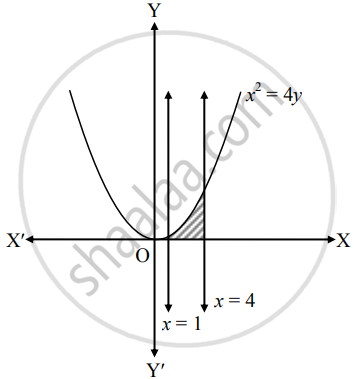# Find the area of the region bounded by the parabola x2 = 4y and The X-axis and the line x = 1, x = 4 - Mathematics and Statistics

Sum

Find the area of the region bounded by the parabola x2 = 4y and The X-axis and the line x = 1, x = 4

#### Solution

Given equation of the parabola is x2 = 4y.Required area = int_1^4 y  "d"x

= int_1^4 x^2/4  "d"x

= 1/4[x^3/3]_1^4

= 1/12(4^3 - 1^3)

= 1/12(64 - 1)

= 63/12

= 21/4 sq.units

Concept: Area Bounded by the Curve, Axis and Line
Is there an error in this question or solution?